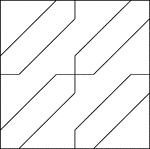Geometric Block Pattern 61

Geometric pattern for translation and rotation exercises.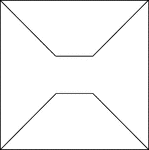Geometric Block Pattern 62

Geometric pattern for translation and rotation exercises.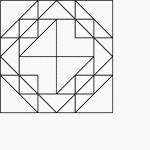Geometric Block Pattern 63

Geometric pattern for translation and rotation exercises.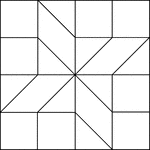Geometric Block Pattern 64

Geometric pattern for translation and rotation exercises.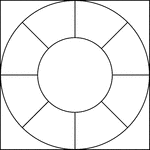Geometric Block Pattern 66

Geometric pattern for translation and rotation exercises.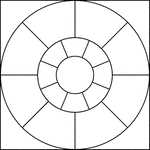Geometric Block Pattern 67

Geometric pattern for translation and rotation exercises.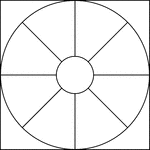Geometric Block Pattern 68

Geometric pattern for translation and rotation exercises.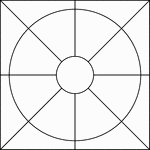Geometric Block Pattern 69

Geometric pattern for translation and rotation exercises.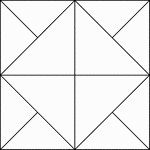Geometric Block Pattern 7

Geometric pattern for translation and rotation exercises.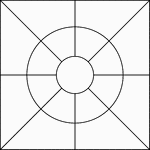Geometric Block Pattern 70

Geometric pattern for translation and rotation exercises.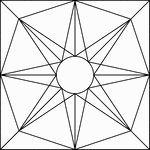Geometric Block Pattern 71

Geometric pattern for translation and rotation exercises.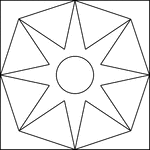Geometric Block Pattern 72

Geometric pattern for translation and rotation exercises.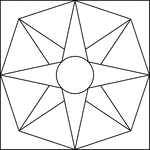Geometric Block Pattern 73

Geometric pattern for translation and rotation exercises.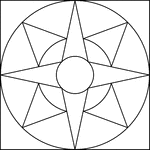Geometric Block Pattern 74

Geometric pattern for translation and rotation exercises.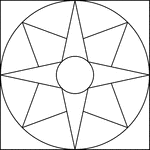Geometric Block Pattern 75

Geometric pattern for translation and rotation exercises.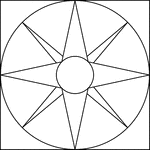Geometric Block Pattern 76

Geometric pattern for translation and rotation exercises.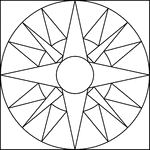Geometric Block Pattern 77

Geometric pattern for translation and rotation exercises.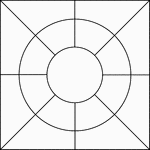Geometric Block Pattern 78

Geometric pattern for translation and rotation exercises.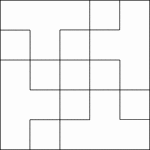Geometric Block Pattern 79

Geometric pattern for translation and rotation exercises.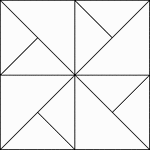Geometric Block Pattern 8

Geometric pattern for translation and rotation exercises.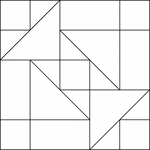Geometric Block Pattern 80

Geometric pattern for translation and rotation exercises.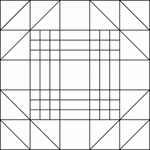Geometric Block Pattern 81

Geometric pattern for translation and rotation exercises.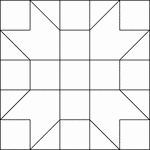Geometric Block Pattern 82

Geometric pattern for translation and rotation exercises.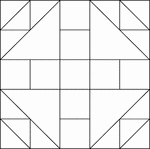Geometric Block Pattern 83

Geometric pattern for translation and rotation exercises.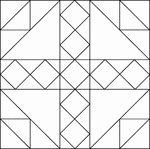Geometric Block Pattern 84

Geometric pattern for translation and rotation exercises.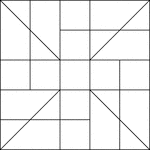Geometric Block Pattern 85

Geometric pattern for translation and rotation exercises.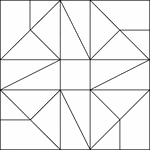Geometric Block Pattern 86

Geometric pattern for translation and rotation exercises.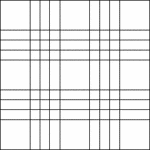Geometric Block Pattern 87

Geometric pattern for translation and rotation exercises.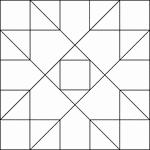Geometric Block Pattern 88

Geometric pattern for translation and rotation exercises.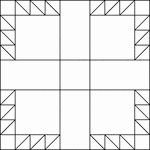Geometric Block Pattern 89

Geometric pattern for translation and rotation exercises.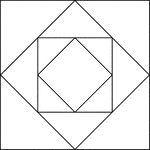Geometric Block Pattern 9

Geometric pattern for translation and rotation exercises.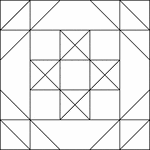Geometric Block Pattern 90

Geometric pattern for translation and rotation exercises.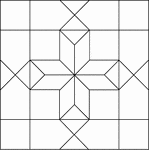Geometric Block Pattern 91

Geometric pattern for translation and rotation exercises.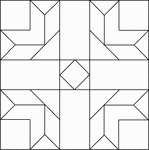Geometric Block Pattern 92

Geometric pattern for translation and rotation exercises.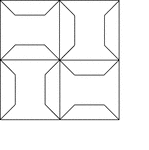Geometric Block Pattern 93

Geometric pattern for translation and rotation exercises.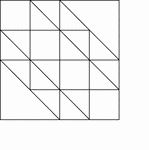Geometric Block Pattern 94

Geometric pattern for translation and rotation exercises.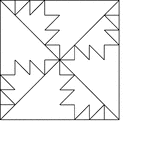Geometric Block Pattern 95

Geometric pattern for translation and rotation exercises.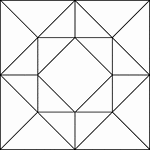Geometric Block Pattern 96

Geometric pattern for translation and rotation exercises.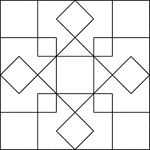Geometric Block Pattern 97

Geometric pattern for translation and rotation exercises.Geometric Block Pattern 98

Geometric pattern for translation and rotation exercises.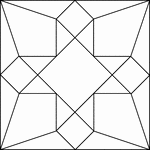Geometric Block Pattern 99

Geometric pattern for translation and rotation exercises.